# 1、继承中的构造和析构的顺序#千锋教育#

class Base
{
public:
Base()
{
cout<<"父类的无参构造函数"<<endl;
}
~Base()
{
cout<<"父类中的析构函数"<<endl;
}
};
class Son:public Base
{
public:
Son()
{
cout<<"子类的无参构造"<<endl;
}
~Son()
{
cout<<"子类中的析构函数"<<endl;
}
};
void test01()
{
Son ob1;
}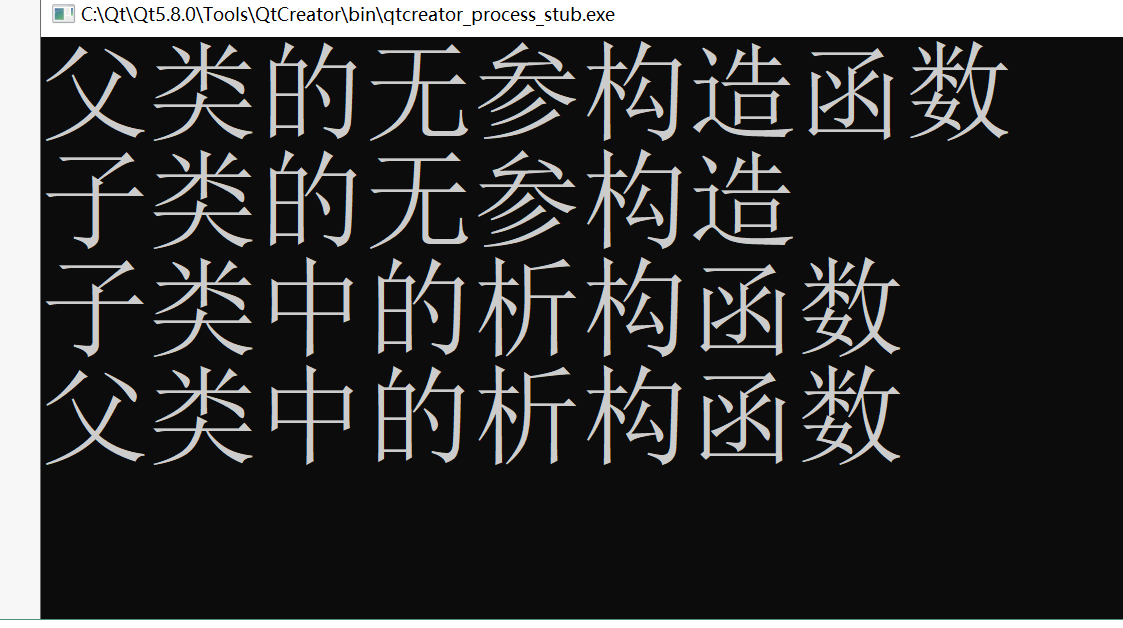## 总结：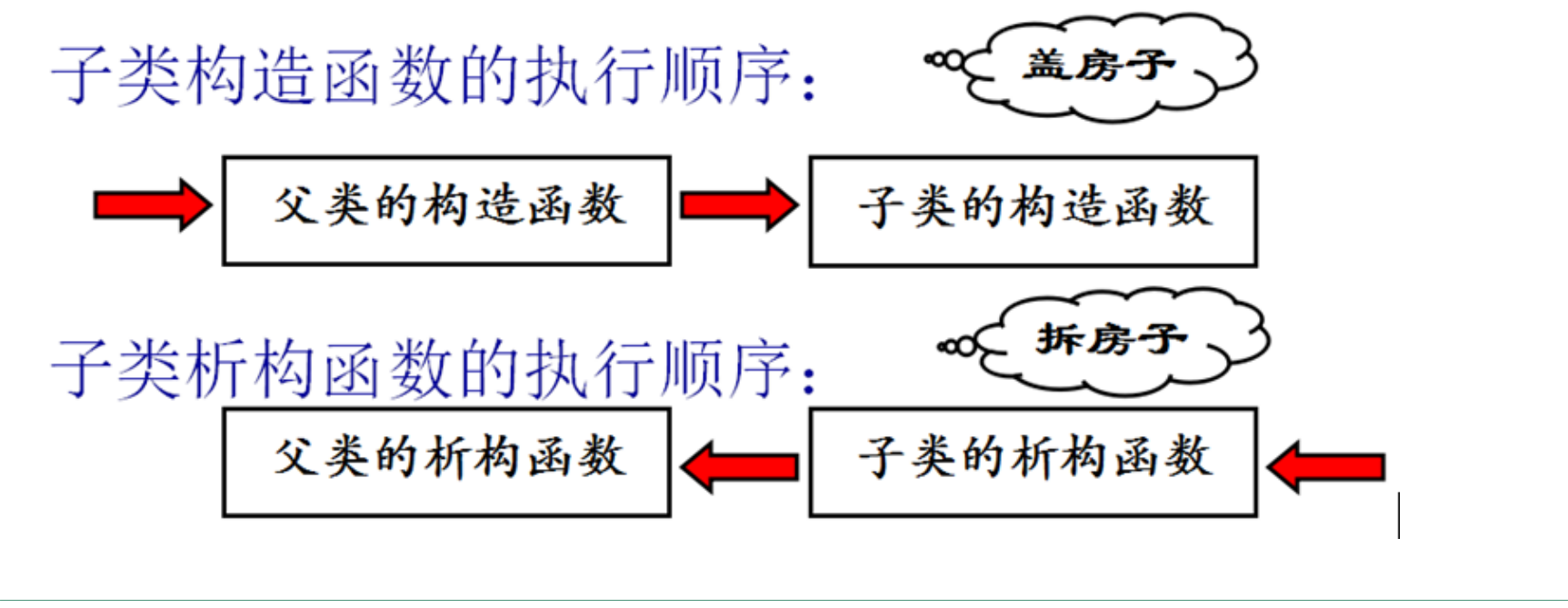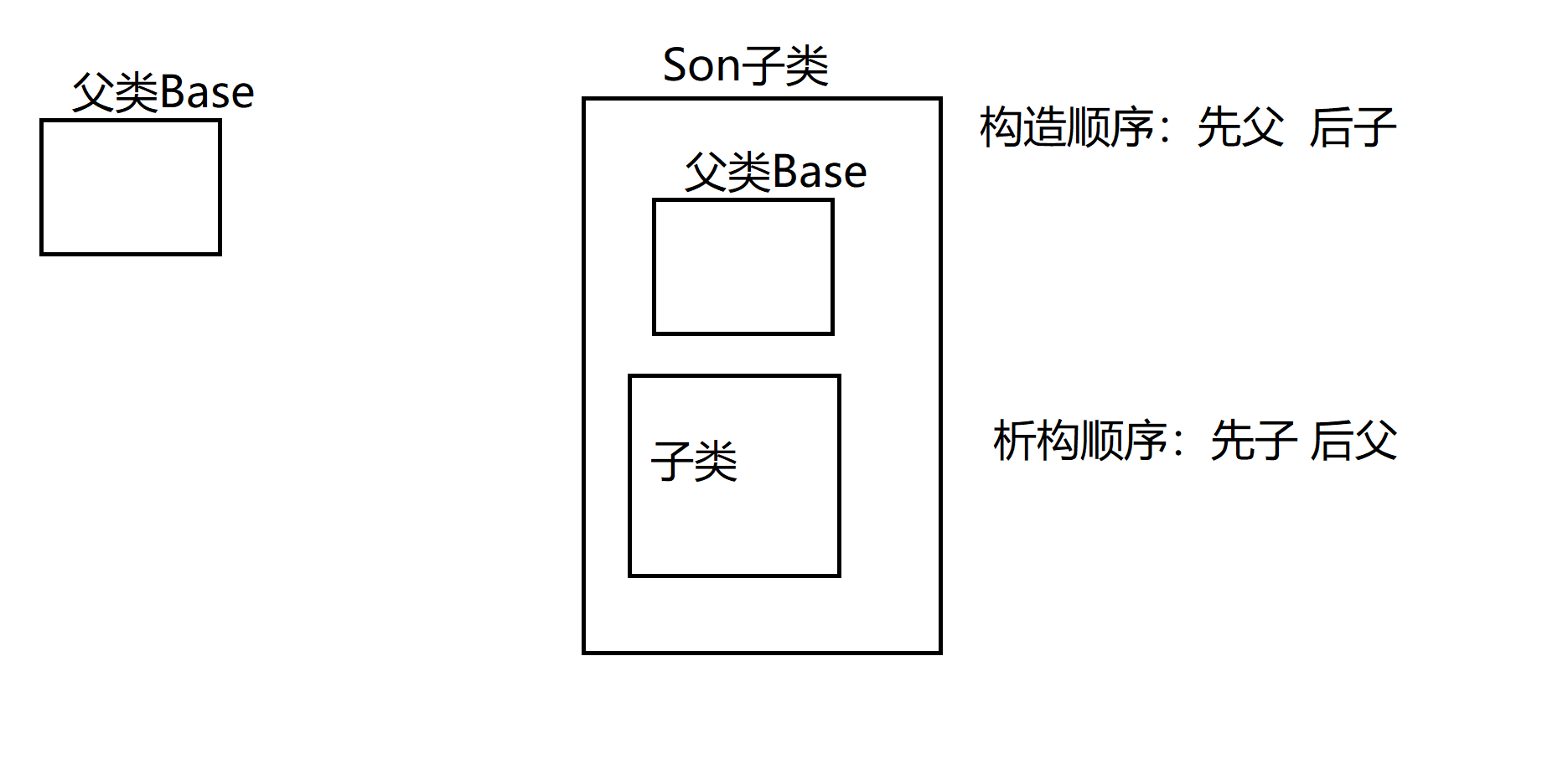# 2、子类中 有父类、对象成员 构造和析构的顺序

class Other
{
public:
Other()
{
cout<<"对象成员的构造函数"<<endl;
}
~Other()
{
cout<<"对象成员的析构函数"<<endl;
}
};
class Base
{
public:
Base()
{
cout<<"父类的无参构造函数"<<endl;
}
~Base()
{
cout<<"父类中的析构函数"<<endl;
}
};
class Son:public Base
{
public:
Son()
{
cout<<"子类的无参构造"<<endl;
}
~Son()
{
cout<<"子类中的析构函数"<<endl;
}

Other ob;//对象成员

};
void test01()
{
Son ob1;
}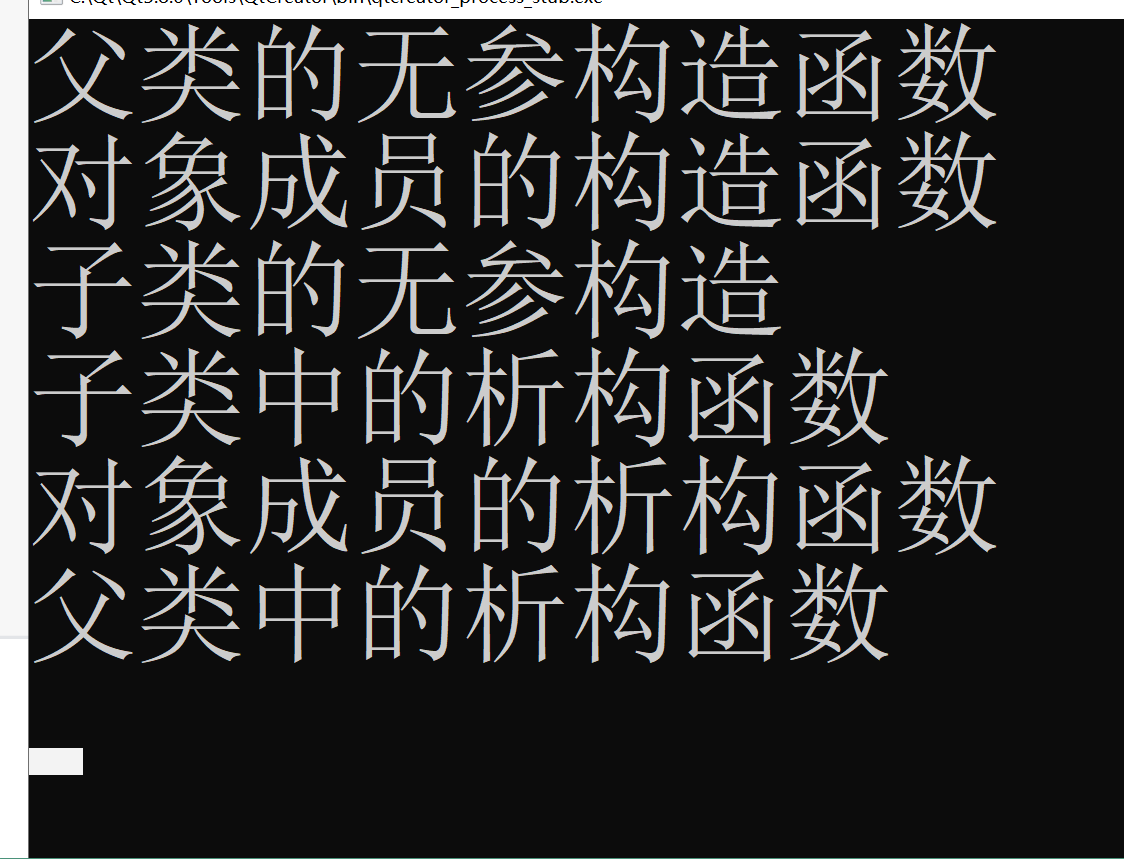## 总结：（重要）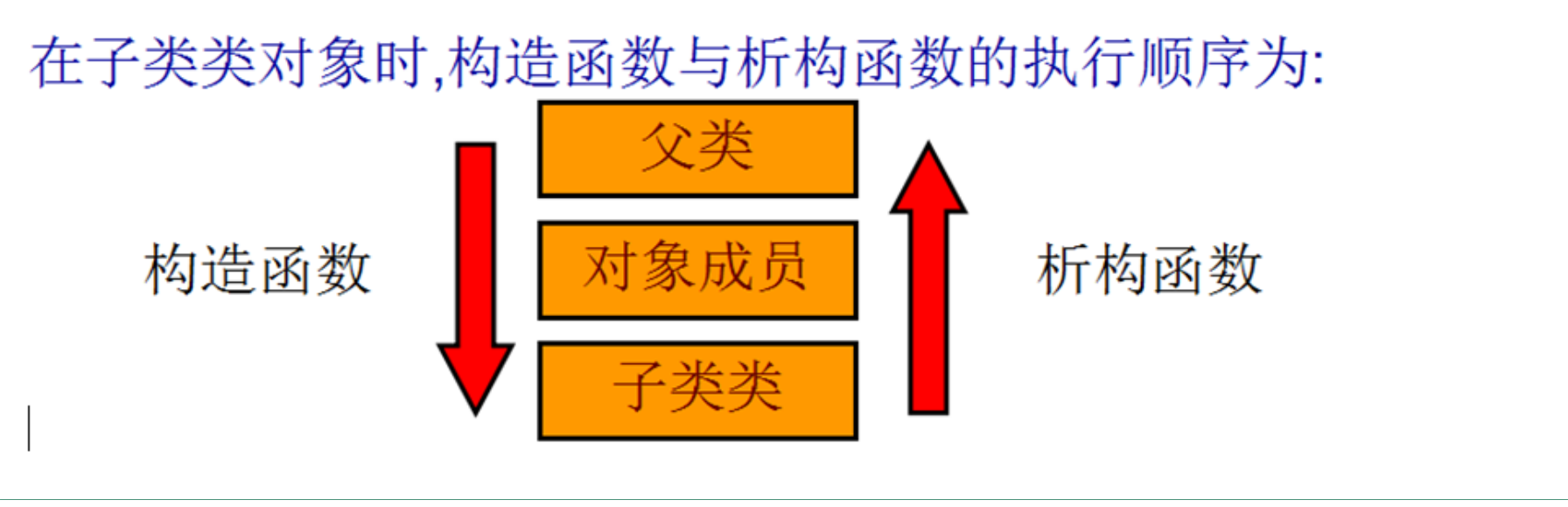# 3、详解 子类中的构造

1、子类会默认调用  父类的 无参构造
2、子类 必须显示 使用初始化列表  调用 父类的有参构造


Son(int a,int b):Base(a),b(b)
{
//this->b = b;
}

class Base
{
private:
int a;
public:

Base()
{
cout<<"父类的无参构造函数"<<endl;
}
Base(int a)
{
this->a = a;
cout<<"父类的有参构造函数"<<endl;
}
~Base()
{
cout<<"父类中的析构函数"<<endl;
}
};
class Son:public Base
{
private:
int b;
public:
Son()
{
cout<<"子类的无参构造"<<endl;
}
Son(int b)
{
this->b = b;
cout<<"子类的有参构造函数int"<<endl;
}

//子类必须用 初始化列表 显示的调用父类的有参构造
//父类名称(参数)
Son(int a,int b):Base(a)//显示的调用父类的有参构造
{
this->b = b;
cout<<"子类的有参构造函数 int int"<<endl;
}
~Son()
{
cout<<"子类中的析构函数"<<endl;
}
};
void test01()
{
//子类 默认 会调用 父类的无参构造
//Son ob1(10);

//子类必须用 初始化列表 显示的调用父类的有参构造
//父类名称+()
Son ob2(10,20);

}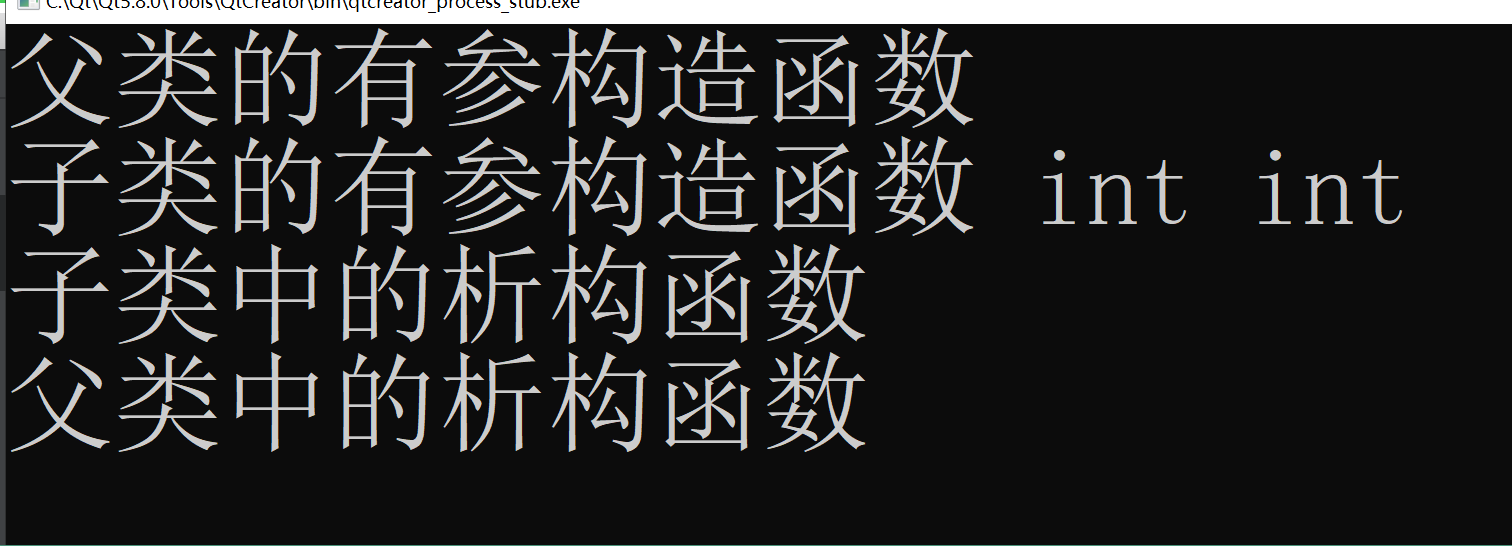## 案例提高：

 Base(int a, int data)
{
this->a = a;
this->data = data;
cout<<"父类的有参构造函数"<<endl;
}


//子类必须用 初始化列表 显示的调用父类的有参构造
//父类名称(参数)
Son(int a,int b, int c):Base(a,c),b(b)//显示的调用父类的有参构造
{
//this->b = b;
cout<<"子类的有参构造函数 int int"<<endl;
}


# 4、父类和子类的同名 成员变量 处理

## 4.2、如果在子类中 必须使用父类中的同名成员 必须加上父类的作用域。

class Base
{
//父类的私有数据 一旦涉及继承 在子类中不可见
public:
int num;
public:
Base(int num)
{
this->num = num;
cout<<"Base有参构造int"<<endl;
}
~Base()
{
cout<<"析构函数"<<endl;
}
};

class Son:public Base
{
private:
int num;
public:
Son(int num1,int num2):Base(num1)
{
this->num = num2;
cout<<"有参构造int int"<<endl;
}

~Son()
{
cout<<"析构函数"<<endl;
}
void showNum(void)
{
//如果在子类中 必须使用父类中的同名成员  必须加上父类的作用域
cout<<"父类中的num = "<<Base::num<<endl;

//当 父类和子类 成员变量同名时  在子类就近原则 选择本作用域的子类成员
cout<<"子类中的num = "<<num<<endl;
}
};

void test01()
{
Son ob1(10,20);
ob1.showNum();
}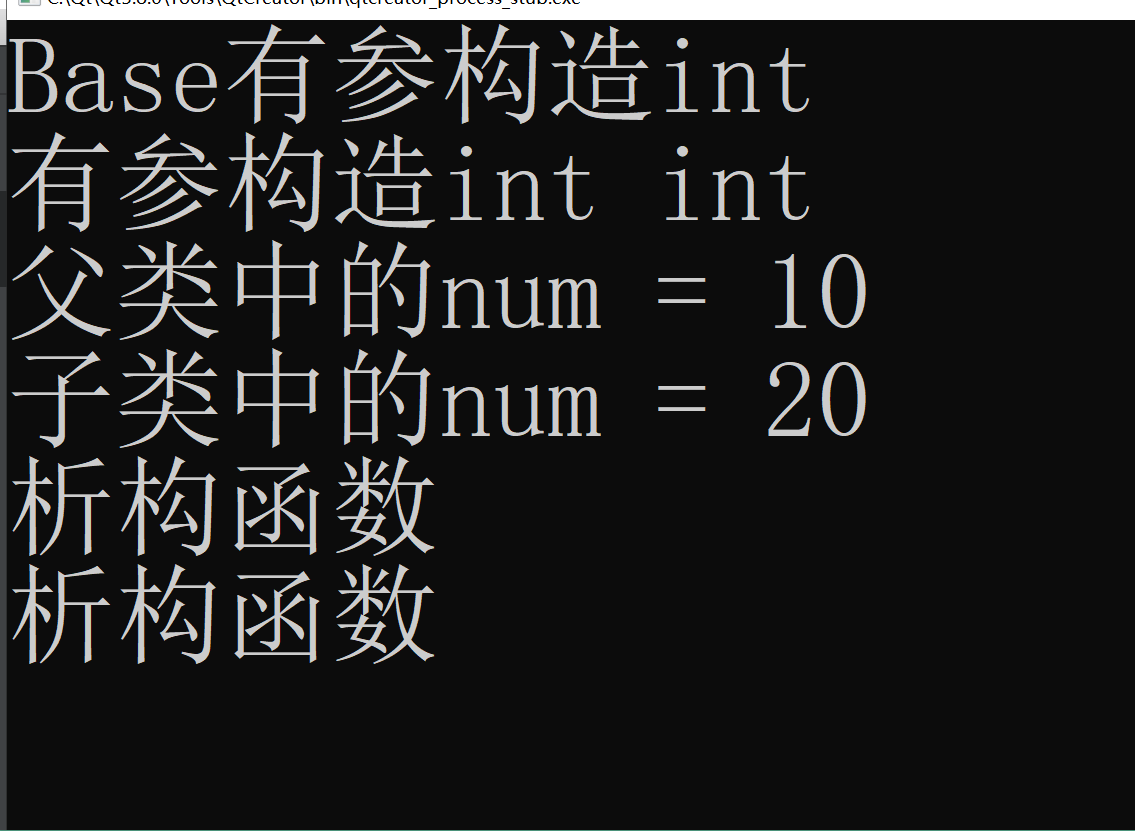## 4.3、子类可以借助 父类的公有方法 间接的操作 父类的私有数据（不可见的数据）

class Base
{

private:
int num;//父类的私有数据 一旦涉及继承 在子类中不可见
public:
Base(int num)
{
this->num = num;
cout<<"Base有参构造int"<<endl;
}
~Base()
{
cout<<"析构函数"<<endl;
}
int getNum(void)
{
return num;
}
};

class Son:public Base
{
private:
int num;
public:
Son(int num1,int num2):Base(num1)
{
this->num = num2;
cout<<"有参构造int int"<<endl;
}

~Son()
{
cout<<"析构函数"<<endl;
}
void showNum(void)
{
//如果在子类中 必须使用父类中的同名成员  必须加上父类的作用域
cout<<"父类中的num = "<<getNum()<<endl;

//当 父类和子类 成员变量同名时  在子类就近原则 选择本作用域的子类成员
cout<<"子类中的num = "<<num<<endl;
}
};

void test01()
{
Son ob1(10,20);
ob1.showNum();
}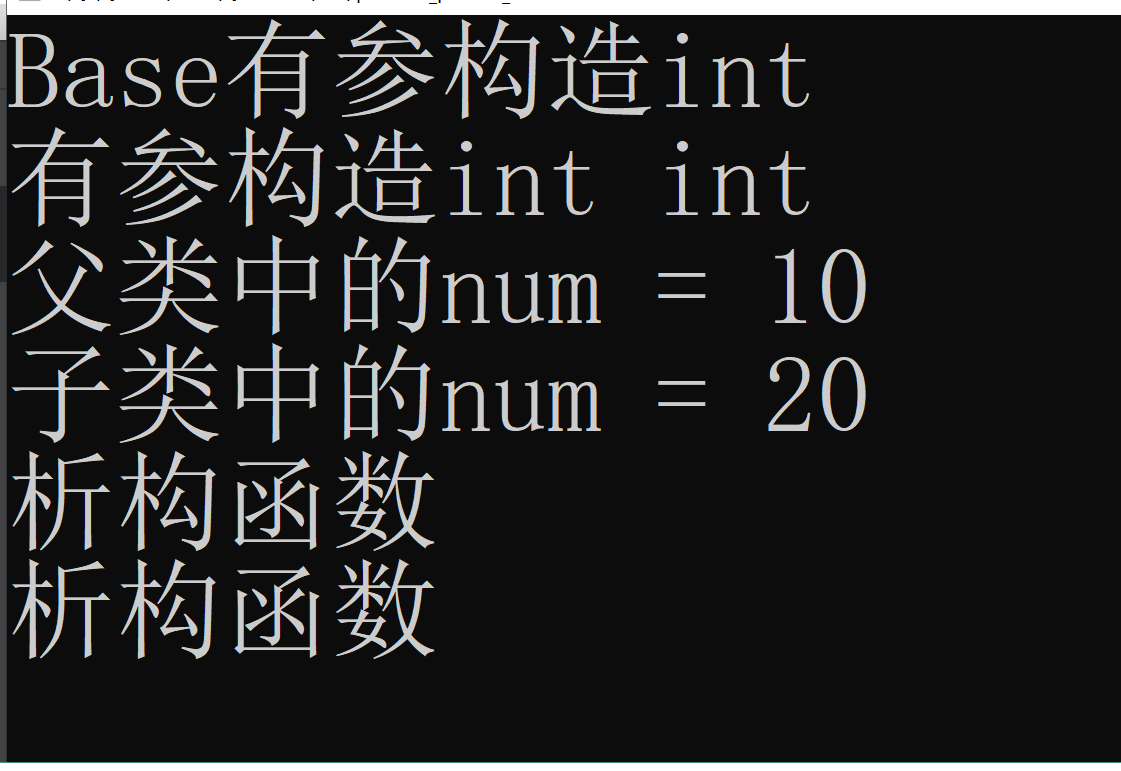# 5、父类和子类的同名 成员函数 处理

## 案例：1子类继承父类所有成员函数 和成员变量

class Base
{
public:
void func(void)
{
cout<<"父类中的void func"<<endl;
}
void func(int a)
{
cout<<"父类中的int func a = "<<a<<endl;
}
};

class Son:public Base
{
public:

};

void test01()
{
//为啥构造和析构除外?父类的构造和析构 只有父类自己知道该怎么做（构造和析构 系统自动调用）
//子类会继承父类所有成员函数(构造和析构函数除外) 和成员变量
Son ob1;
ob1.func();//访问的是父类的void func(void)
ob1.func(10);//访问的是父类的func(int a)
}


## 案例：2子类和父类 同名成员函数

class Base
{
public:
void func(void)
{
cout<<"父类中的void func"<<endl;
}
void func(int a)
{
cout<<"父类中的int func a = "<<a<<endl;
}
};

class Son:public Base
{
public:
//一旦子类 实现了 父类的同名成员函数 将屏蔽所有父类同名成员函数
void func(void)
{
cout<<"子类中voidfunc"<<endl;
}
};

void test01()
{
//为啥构造和析构除外?父类的构造和析构 只有父类自己知道该怎么做（构造和析构 系统自动调用）
//子类会继承父类所有成员函数(构造和析构函数除外) 和成员变量
Son ob1;
ob1.func();
//ob1.func(10);//err //一旦子类 实现了 父类的同名成员函数 将屏蔽所有父类同名成员函数

//如果用户 必须要调用父类 的同名成员函数 必须加作用域
ob1.Base::func();//调用父类的void func
ob1.Base::func(10);//调用父类的int func
}
int main(int argc, char *argv[])
{
test01();
return 0;
}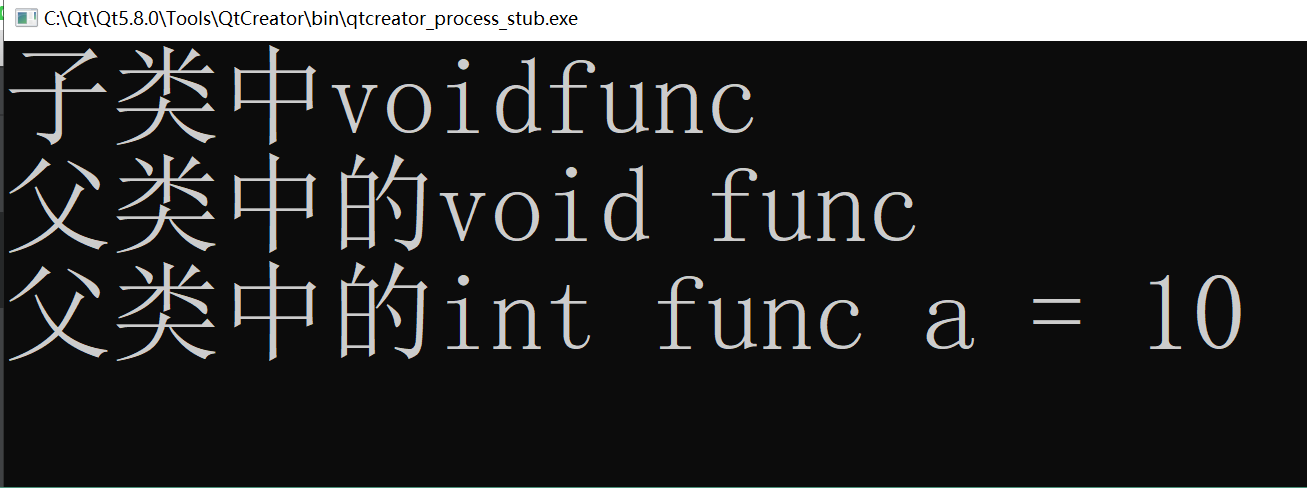# 6、继承中的静态成员特性（了解）

class Base
{
public:
//静态成员属于类 而不属于对象
static int num;
static int data;

static void showData(void);

};
int Base::num  = 100;
int Base::data = 200;

class Son:public Base
{
public:
static int data;//父和子类 静态成员 同名
static void showData(void);
};
int Son::data = 300;

void test01()
{
//从Base类中访问
cout<<Base::num<<endl;

// Son 也拥有了静态成员num
cout<<Son::num<<endl;

//父和子类 静态成员 同名 在子类中 访问子类中的成员
cout<<Son::data<<endl;//200

//父和子类 静态成员 同名 访问父类中的成员 必须加 Base::
cout<<Son::Base::data<<endl;//200

//父和子类 同名静态成员函数 子类默认访问子类的静态成员函数
Son::showData();
//父和子类 同名静态成员函数 子类访问父类的静态成员函数 必须加 Base::
Son::Base::showData();
}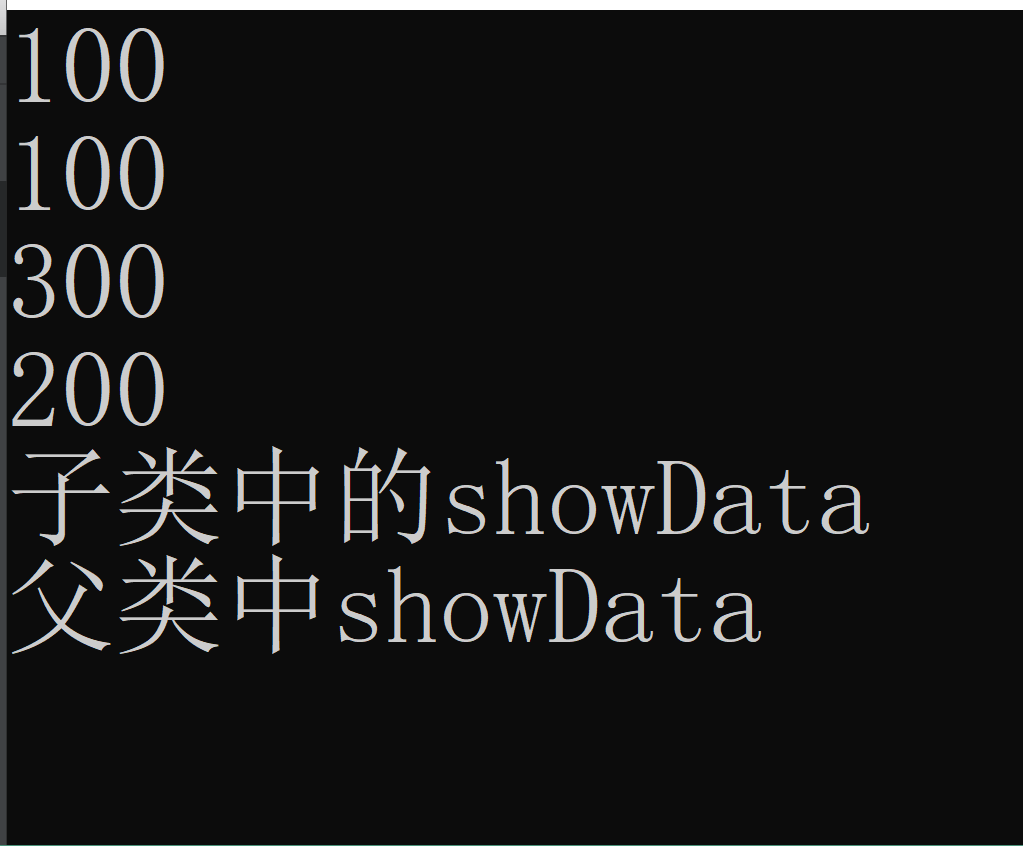# 7、多继承（了解）

## 7.1、多继承的格式：

class 子类: 继承方式1 父类名1,继承方式2 父类名2,继承方式3 父类名3,....
{

};
//表示子类 是由 父类名1,父类名2,父类名3...共同派生出来

class Base1
{
public:
int a;
};
class Base2
{
public:
int b;
};

class Son:public Base1,public Base2
{
//Son类 拥有了a  b
};
int main(int argc, char *argv[])
{
Son ob;
ob.a = 100;
ob.b = 200;
return 0;
}


## 7.2、多继承容易产生二义性： （解决办法1 使用作用域）

class Base1
{
public:
int a;
};
class Base2
{
public:
int a;
};

class Son:public Base1,public Base2
{

};
int main(int argc, char *argv[])
{
Son ob;
//ob.a = 100;//err Base1 和 Base2中都有a成员同名
//解决办法:加作用域
ob.Base1::a = 100;
ob.Base2::a = 200;
return 0;
}


## 7.3、菱形继承（具有公共祖先 的多继承）

class Animal
{
public:
int data;
};

class Sheep:public Animal
{
public:
};
class Tuo:public Animal
{
public:
};

class SheepTuo:public Sheep,public Tuo
{
public:
};
int main(int argc, char *argv[])
{
SheepTuo st;
//SheepTuo 从Sheep中继承data  从Tuo继承data 就产生二义性
//st.data = 200;//err
//第一中方式：加作用域解决
st.Sheep::data = 200;
st.Tuo::data = 300;

return 0;
}


# 8、普通继承：vs studio分析

class Animal
{
public:
int data;
};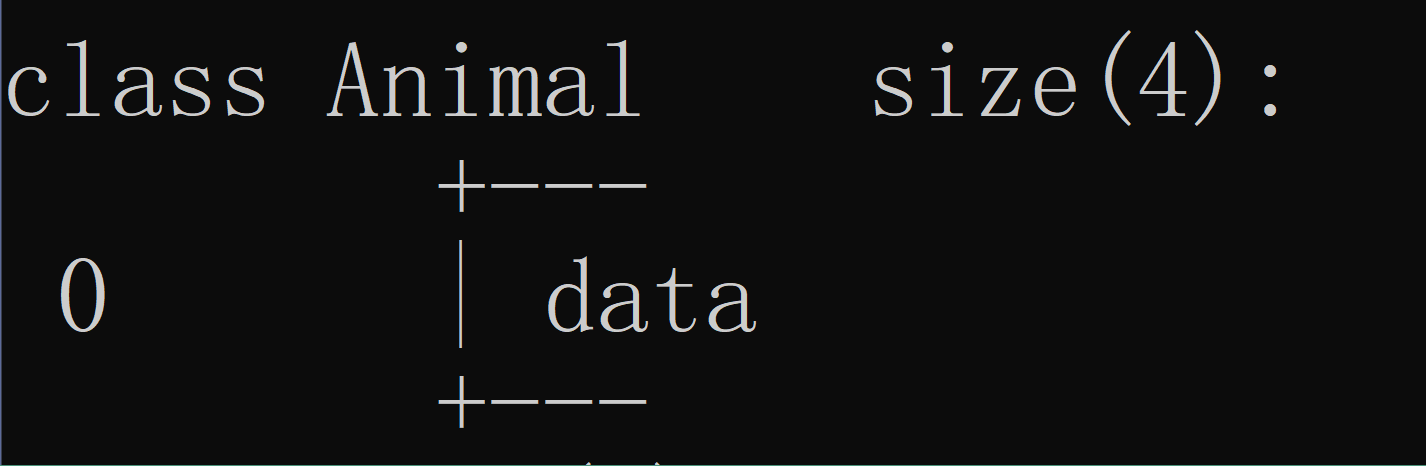class Sheep:public Animal
{
public:
};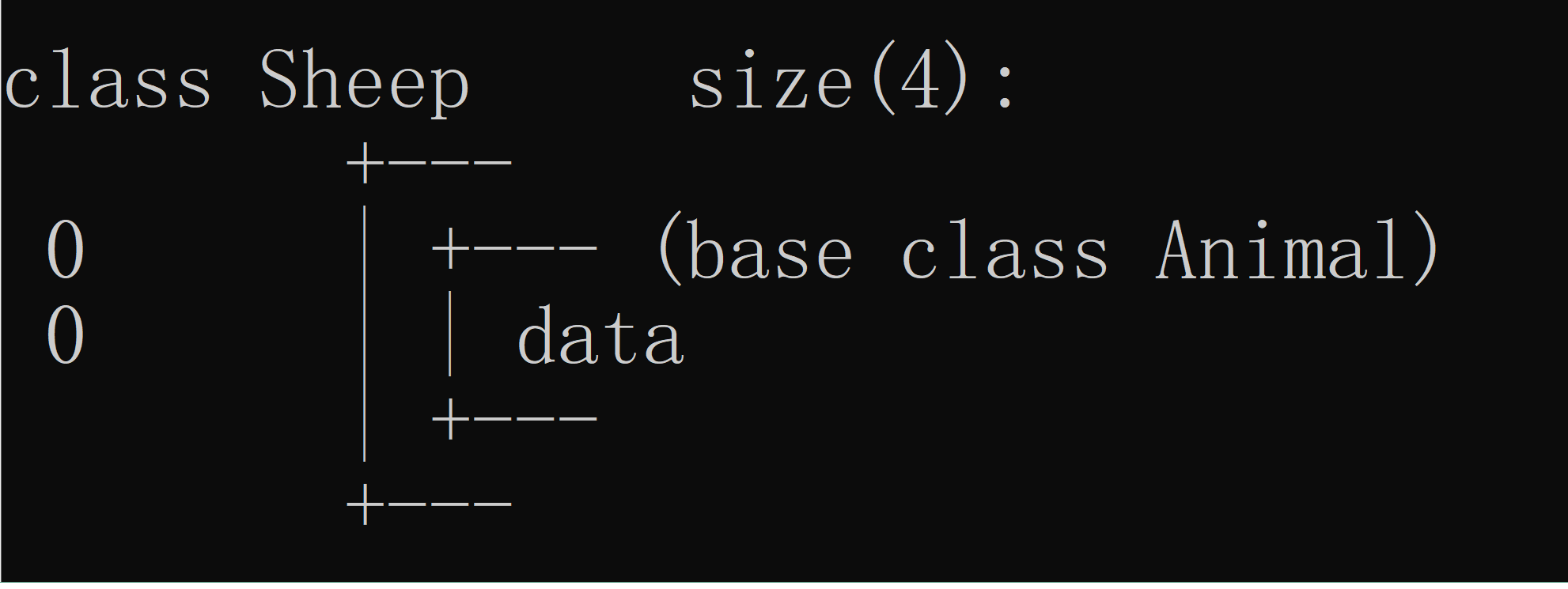class Tuo:public Animal
{
public:
};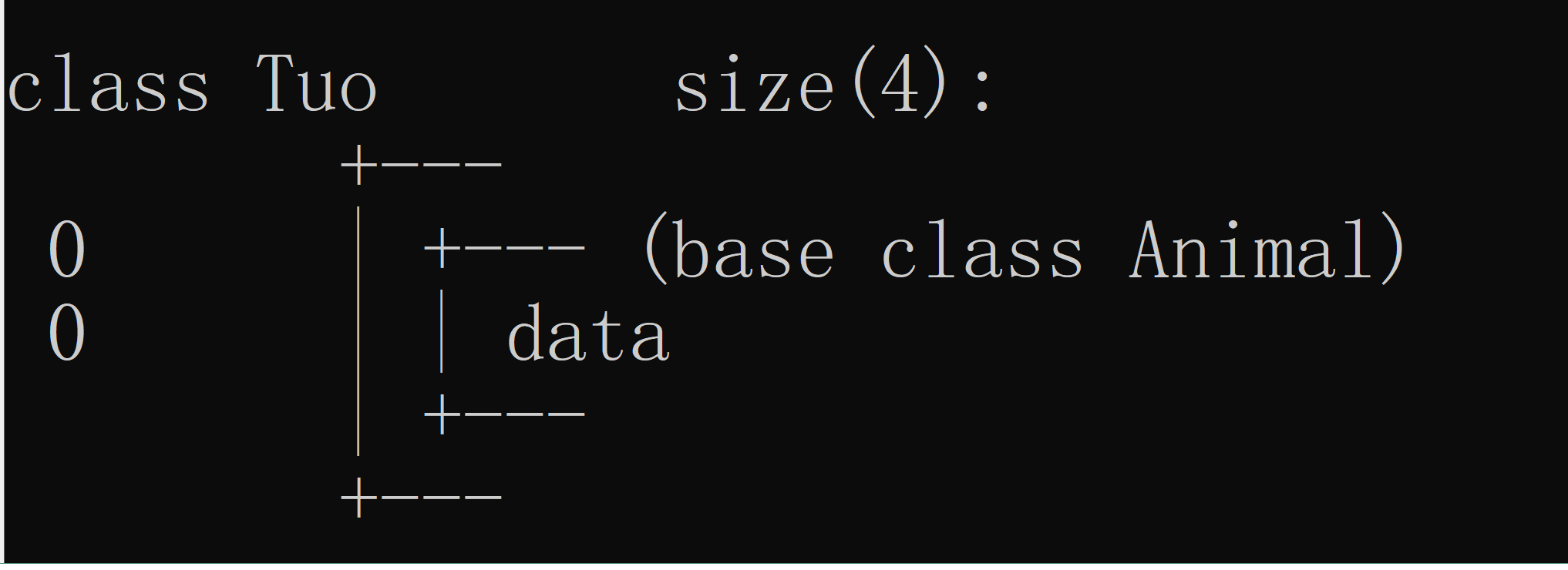class SheepTuo:public Sheep,public Tuo
{
public:
};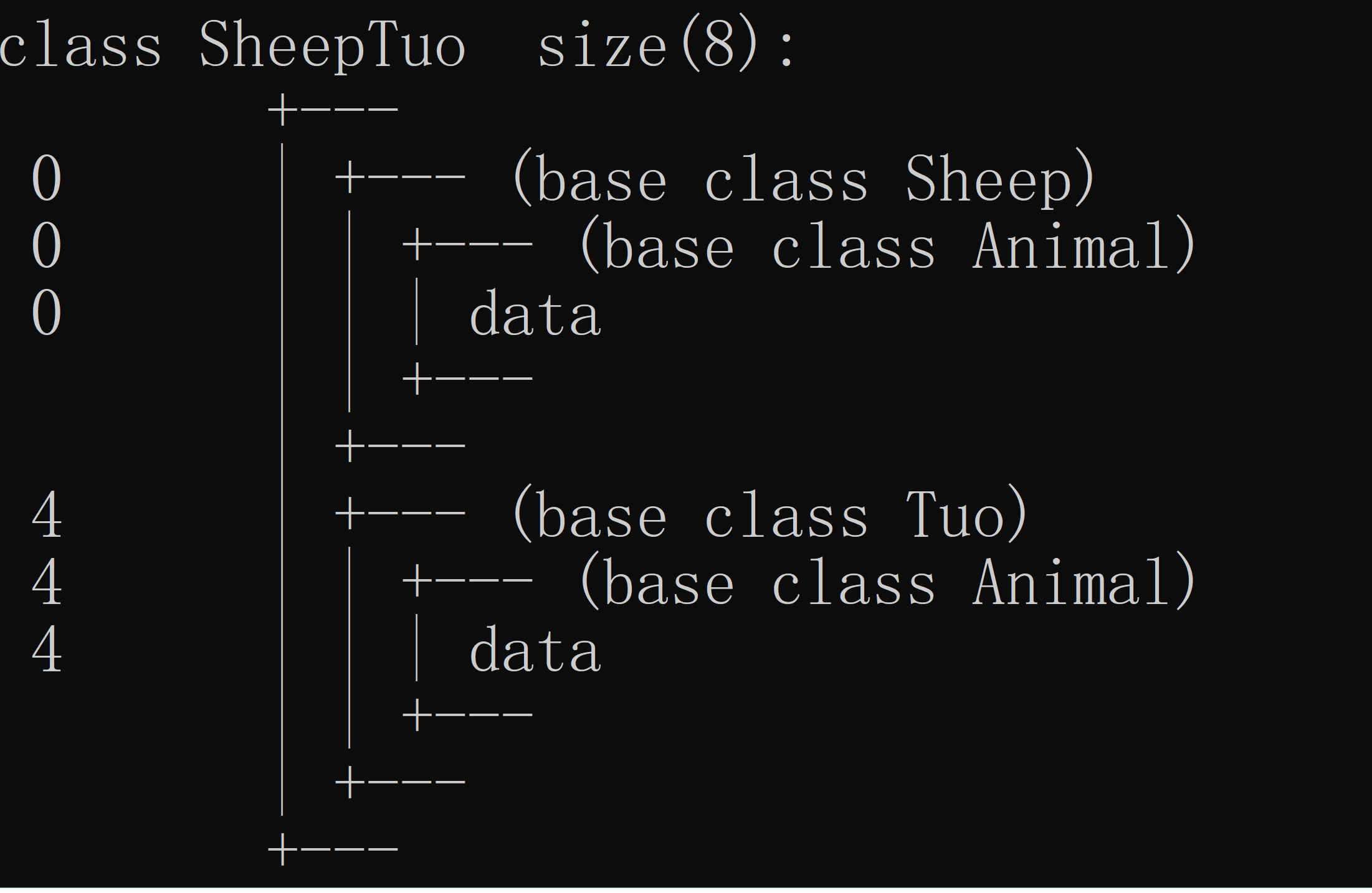# 9、虚继承（了解） vs studio分析

virtual修饰继承方式

//继承的动作 虚继承
//父类：虚基类
class 子类:virtual public 父类
{
};


class Animal
{
public:
int data;
};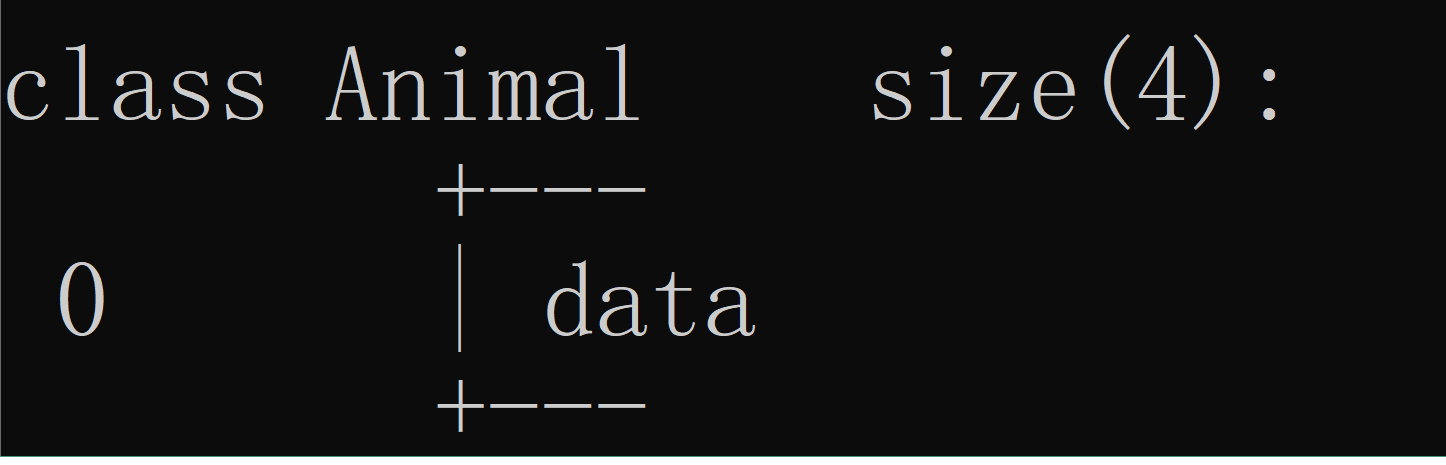class Sheep:virtual public Animal
{
public:
};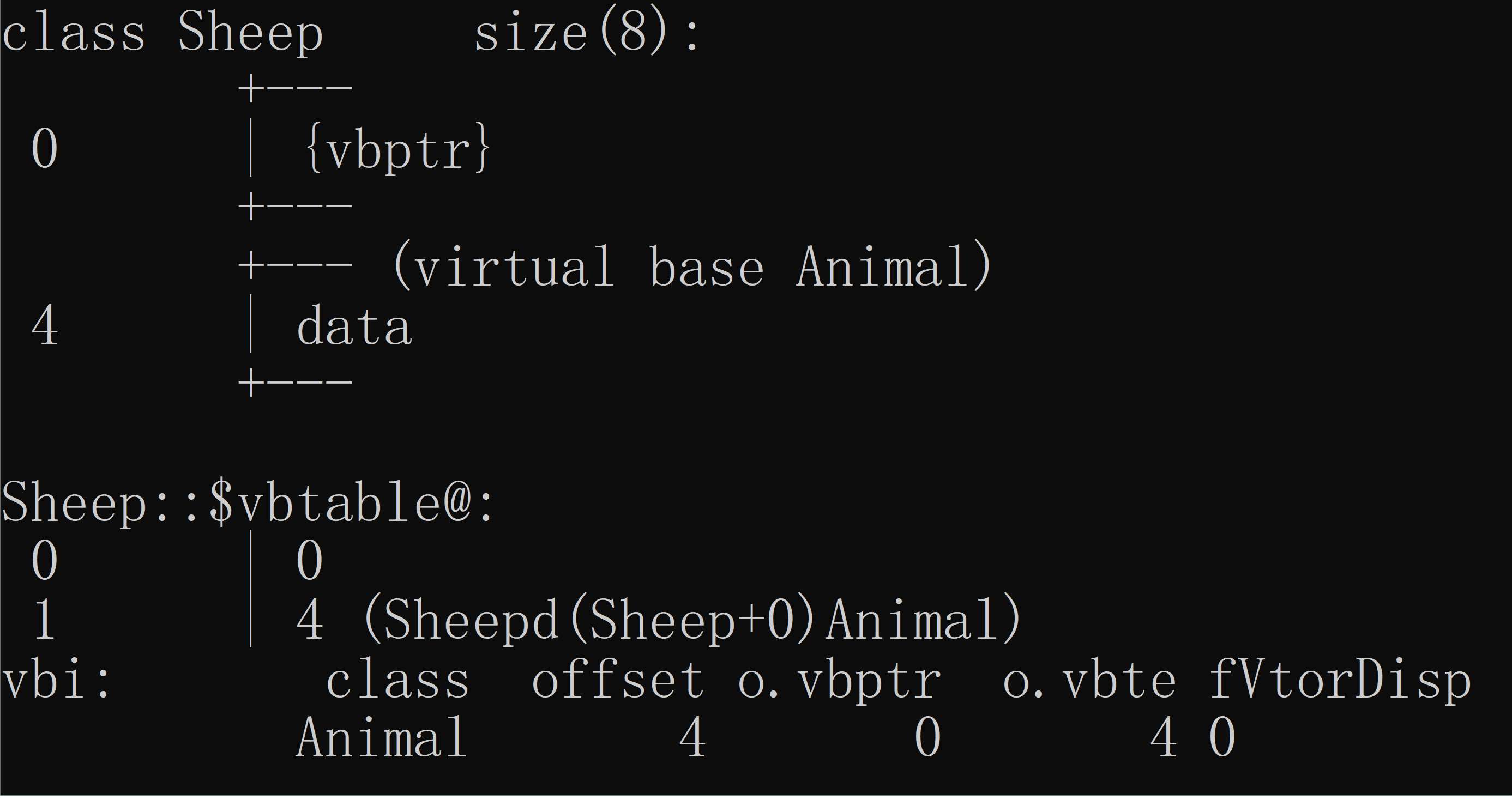vbptr（虚基类指针） 其中v是virtual 虚 b是base 基类 prt指针
（vbptr指向虚基类表）
vbtable(虚基类表 ） 保存了当前的虚指针相对于虚基类的首地址的偏移量

class Tuo:virtual public Animal
{
public:
};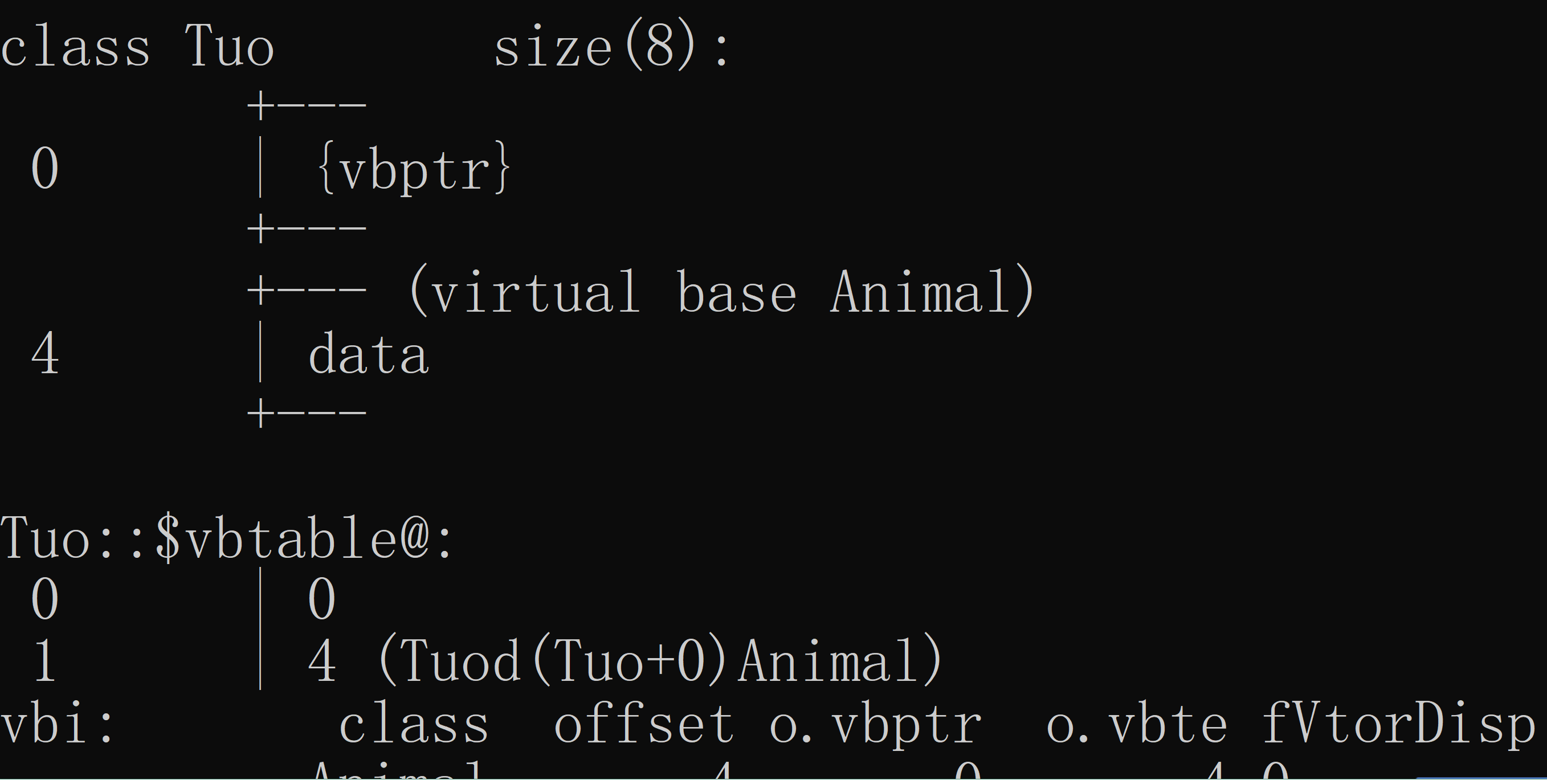class SheepTuo:public Sheep,public Tuo
{
public:
};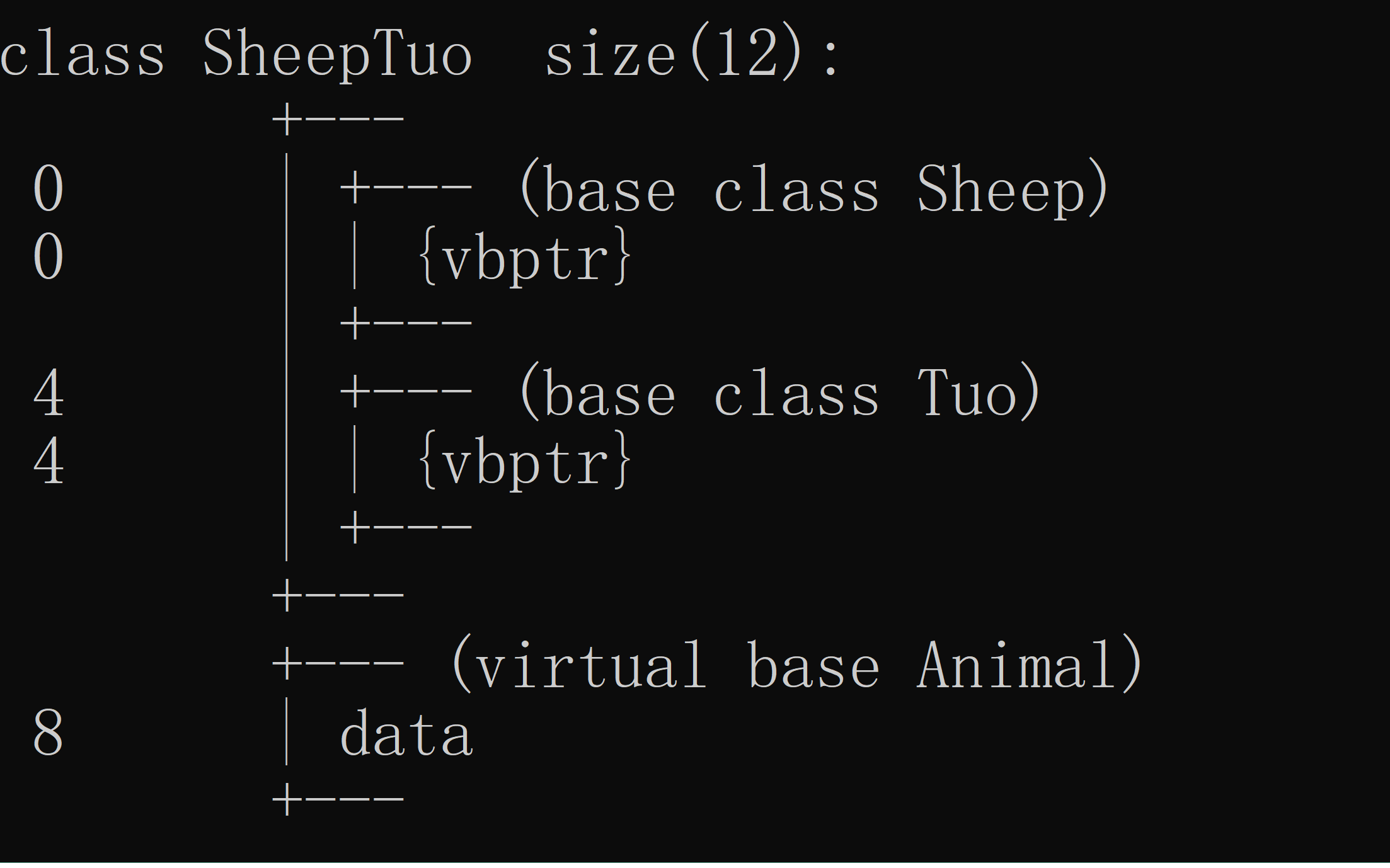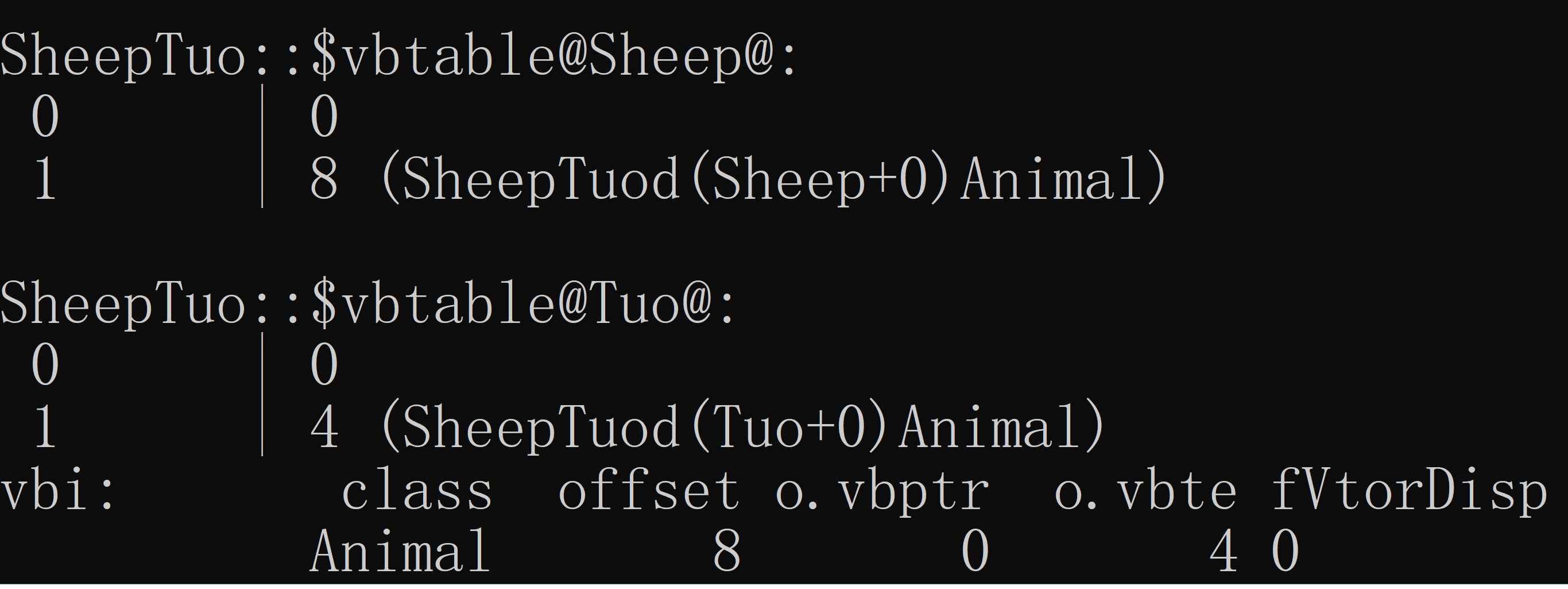## 案例1：

#define _CRT_SECURE_NO_WARNINGS
#include <iostream>
#include<string.h>
using namespace std;

class Animal
{
public:
int data;
};

class Sheep :virtual public Animal
{
public:
};
class Tuo :virtual public Animal
{
public:
};

class SheepTuo :public Sheep, public Tuo
{
public:
};
int main(int argc, char* argv[])
{
SheepTuo st;
st.data = 200;

//通过Sheep的vbptr 寻找vbptr距离虚基类首地址的偏移量
//&st == vbptr
//*(int *)&st sheep 的虚基类表的起始位置
int off_set = (int)*((int*)(*(int*)&st) + 1);
cout << off_set << endl;

//通过sheep的vbptr 和 off_set定位虚基类的首地址
cout << ((Animal*)((char*)&st + off_set))->data << endl;

return 0;
}©️2019 CSDN 皮肤主题: 1024 设计师: 上身试试